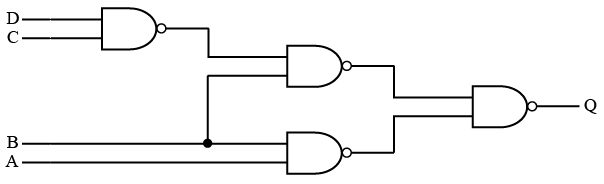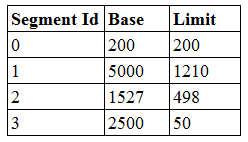# ISRO Scientist or Engineer Computer Science 2014

Instructions

For the following questions answer them individually

Question 11

# An aggregation association is drawn using which symbol?Question 12

# How manystates are there in a minimum state deterministic finite automaton accepting the language $$L = \left\{w \mid w \in (0, 1)^*\right\}$$, {number of 0’s is divisible by 2 and numberof 1's is divisible by 5, respectively} ?Question 13

# Which of the following is TRUE with respect to a Reference?Question 14

# There are 200 tracks on a disk platter and the pending requests have comein the order — 36, 69, 167, 76, 42, 51, 126, 12, and 199. Assume the arm is located at the $$100^{th}$$ track and moving towardstrack 200. If sequence of disc access is 126, 167, 199, 12, 36, 42, 51, 69, and 76 then which disc access scheduling policy is used?Question 15

# Consider the logic circuit given below:Q = ...........?Question 16

# What is routing algorithm used by OSPF routing protocol?Question 17

# If each address space represents one byte of storage space, how many address lines are needed to access RAM chips arranged in a $$4 \times 6$$ array, where each chip is $$8K \times 4$$ bits?Question 18

# Consider the following segment table in segmentation scheme:What happens if the logical address requested is - Segment Id 2 and Offset 1000?Question 19

# The numberofbit strings of length 8 that will either start with 1 or end with 00 is .............?Question 20

# Which of the following is not a maturity level as per Capability Maturity Model?OR